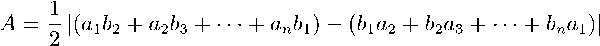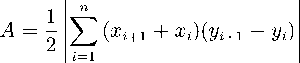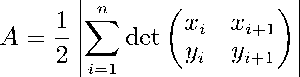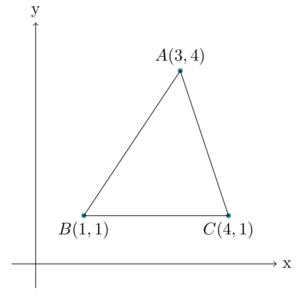×

Search anything:

# Oriented area of a triangle

#### Algorithms computational geometryGet this book -> Problems on Array: For Interviews and Competitive Programming

In this article, we discuss how to find the area of an oriented Polygon using the shoelace algorithm and as an example we find the area of an oriented triangle.

1. Introduction to Oriented Triangle
2. Steps to find area of Polygon
3. Shoelace formula
4. Oriented area of triangle
5. Implementation in C++
6. Time and Space Complexity Analysis

Prerequisite: Shoelace formula

## Introduction to Oriented Triangle

Oriented triangles are just regular triangles but with some orientation and thus have oriented areas.
We can calculate the area of an oriented triangle using the shoelace formula.

The shoelace formula or algorithm is a mathematical algorithm used to determine the area of a simple polygon whose vertices are described by their cartesian coordinates on a plane. In our case we have a triangle.

## Steps to find area of Polygon

1. List all vertices in anticlockwise order in a table structure and note coordinates x and y in two separate columns.
2. Calculate sum by multiplying x coordinate with the y coordinated in the row below. (go back to first row when we reach the bottom).
3. Calculate the sum by mulitplying each y coordinate with x coordinate in the row below. (go back to first row when we reach the bottom).
4. Subtract the second sum from the first sum to get an absolute value.
5. Divide the resulting value by 2, this is the actual area of the polygon.

## Shoelace formula

General formulaIn the form of a summation.In terms of determinants.A - area of polygon.
n - number of sides of the polygon.
(xi yi) where i = 1, 2, ... n, ordered vertices of the polygon(corners).

It is called the shoelace formula because we write all coordinates in a column and begin to compute the cross products by multiplying x1y1 - x2y1, x2y3 - x3y2, it resembles a laced shoe like shown below.## Oriented area of triangle

We are given points p1, p2, p3 and we are to calculate an oriented (signed) area formed by the triangle.

Signed means that the area is positive if the vertices of the triangle are counterclockwise, negative if the vertices are clockwise and zero if the points are collinear.

By using the signed area we can get tha unsigned area and determine if points lie in a clockwise or counterclockwise order.### How to calculate?

We use the fact that the determinant of a 2x2 matrix is equal to the signed area of a parallelogram spanned by column or row vectors of the matrix.
By dividing this area by 2 we get the area of the rectangle which is the result we are interested in.
We use (p1, p2) and (p2, p3) as column vectors to calculate a 2x2 determinant.
Therefore we have

$2S=|\begin{array}{cc}{x}_{2}-{x}_{1}& {x}_{3}-{x}_{2}\\ & \\ {y}_{2}-{y}_{1}& {y}_{3}-{y}_{2}\end{array}|=\left({x}_{2}-{x}_{1}\right)\left({y}_{3}-{y}_{2}\right)-\left({x}_{3}-{x}_{2}\right)\left({y}_{2}-{y}_{1}\right)$

## Implementation in C++

#include<iostream>

using std::vector;
using std::cout;
using std::endl;

class PolygonArea{
public:
float triArea(vector<vector<int>> coords){
float sum1 = 0, sum2 = 0, area = 0;

int n = coords.size();

for(int i = 0; i < n-1; i++){
sum1 = sum1 + coords[i] * coords[i+1];
sum2 = sum2 + coords[i] * coords[i+1];
}
sum1 = sum1 + coords[n-1] * coords;
sum2 = sum2 + coords * coords[n-1];

area = (sum1 - sum2) / 2;
return area;
}
};

int main(){
PolygonArea pa;
vector<vector<int>> triangle = {{3, 4}, {1, 1}, {4, 1}};
cout << pa.triArea(triangle) << endl;
return 0;
}


#### Output.

4.5


## Time and Space Complexity Analysis

For n vertices it takes O(N) multiplications.

The space is constant O(1), we know that a triangle has 3 sides, so we allocate constant memory.

### Applications

• Computer graphics
• Surveying

With this article at OpenGenus, you must have the complete idea of Oriented area of a triangle and algorithm to find it.

#### Erick Lumunge

Erick is a passionate programmer with a computer science background who loves to learn about and use code to impact lives positively.

Oriented area of a triangle